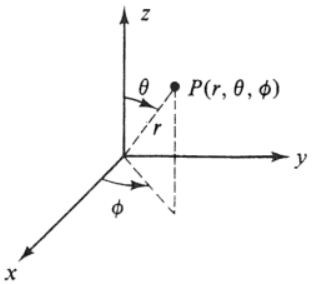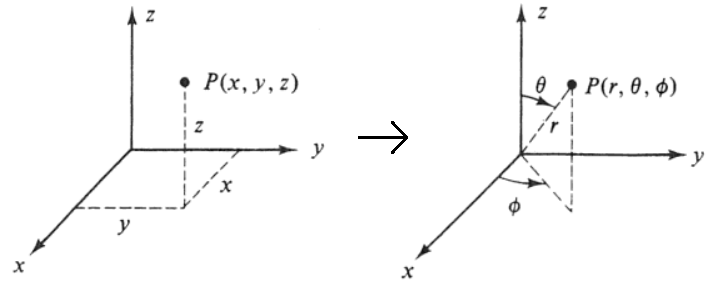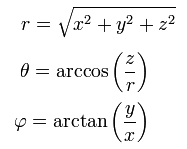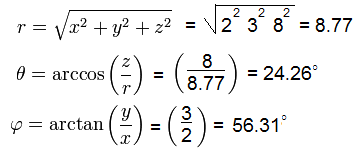﻿ Cartesian to Spherical Coordinates Calculator ﻿# Cartesian to Spherical Coordinates Calculator### Cartesian (Rectangular) to Spherical Coordinates System DiagramX: Y: Z: ° rad

This spherical coordinates converter/calculator converts the rectangular (or cartesian) coordinates of a unit to its equivalent value in spherical coordinates, according to the formulas shown above.

Rectangular coordinates are depicted by 3 values, (X, Y, Z). When converted into spherical coordinates, the new values will be depicted as (r, θ, φ).

To use this calculator, a user just enters in the (X, Y, Z) values of the rectangular coordinates and then clicks the 'Calculate' button, and the spherical coordinates will be automatically computed and shown below.

This calculator can be used to convert 2-dimensional (2D) or 3-dimensional rectangular coordinates to its equivalent spherical coordinates. If desired to convert a 2D rectangular coordinate, then the user just enters values into the X and Y form fields and leaves the 3rd field, the Z field, blank. If desired to convert a 3D rectangular coordinate, then the user enters values into all 3 form fields, X, Y, and Z.

By default, the calculator will compute the result in degrees. However, by using the drop-down menu, the option can changed to radians, so that the result will be computed in radians.

Example Calculations

### Example With Rectangular (Cartesian) Coordinates

Convert the rectangular coordinates (2, 3, 8) into its equivalent spherical coordinates.This answer is calculated in degrees.

Related Resources

﻿